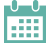# Special Event

New in Wolfram Language 13.1 Webinar Series

Learn about what's new in the latest release of Mathematica and the Wolfram Language in this three-part webinar series. All sessions feature Wolfram experts to discuss what's new in their areas of specialty and answer your questions.

# Interactive Courses

An Elementary Introduction
to the Wolfram Language

Learn the Wolfram Language and modern computational thinking from Stephen Wolfram's book.

Introduction to Cryptography

Learn about the concepts, underlying math and techniques of historic and modern cryptography.

Introduction to Image Processing

Learn the fundamentals of digital image processing along with practical applications using the Wolfram Language.

Introduction to Notebooks

Learn to use Wolfram Notebooks for computing, programming, generating reports and creating presentations with this interactive course.

Learn to chart a path from questions to actionable insights using modern analytical techniques, computation and a flexible, integrated project workflow.

Signals, Systems and Signal Processing

Learn about the concepts, mathematics, principles and techniques of signal processing in linear, time-invariant (LTI) systems.

Visual Explorations in Data Science

Learn about data exploration with visualizations that leverage the richness of the Wolfram Language.

Wolfram Summer School
Lectures 2018

Deepen your understanding of the Wolfram Language and explore topics from the 2018 summer school curriculum.

# Instructor-Led CoursesView all scheduled courses and events. »